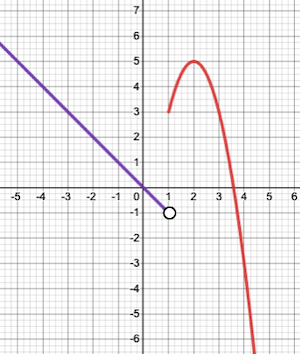# Precalculus - One Sided Limits

## Introduction

• We know that $\underset{x\to a}{\mathrm{lim}}f\left(x\right)$ means that as $x$ "approaches" a real value $a$ (from the left or right side), the function $f\left(x\right)$ "approaches" $L$. A limit expressed in this form is called a two-sided limit.
• There may be cases when we want to find the limits of functions from one side only. Such limits are called one-sided limits.

## Evaluating One-sided limits

Consider the graph of the function $f\left(x\right)$ shown below.We notice that, as $x$ "approaches" $1$ from the left side, $f\left(x\right)$ "approaches" $-1$. Hence, the Left-hand limit of $f\left(x\right)$ close to $x=1$ is -1 or $\underset{x\to {1}^{-}}{\mathrm{lim}}f\left(x\right)=-1$.

Likewise, as $x$ "approaches" 1 from the right side, $f\left(x\right)$ "approaches" $3$. Hence, the Right-hand limit of $f\left(x\right)$ close to $x=1$ is 3 or $\underset{x\to {1}^{+}}{\mathrm{lim}}f\left(x\right)=3$.

Based on the above observations, we can define the left-hand limit and right-hand limit of any function.

Left-hand limit (LHL):

• The behavior of a function $f\left(x\right)$ as $x$ approaches a real value $a$ from the left side is called its left-hand limit.
• Mathematically, it is expressed as $\underset{x\to {a}^{-}}{\mathrm{lim}}f\left(x\right)$.
• To find LHL algebraically, we rewrite the limit as $\underset{x\to {a}^{-}}{\mathrm{lim}}f\left(x\right)=\underset{h\to 0}{\mathrm{lim}}f\left(a-h\right)$ where $h\to 0$ and then evaluate the limit.

Right-hand limit (RHL):

• The behavior of a function $f\left(x\right)$ as $x$ approaches a real value $a$ from the right side is called its right-hand limit.
• Mathematically, it is expressed as $\underset{x\to {a}^{+}}{\mathrm{lim}}f\left(x\right)$.
• To find RHL algebraically, we rewrite the limit as $\underset{x\to {a}^{+}}{\mathrm{lim}}f\left(x\right)=\underset{h\to 0}{\mathrm{lim}}f\left(a+h\right)$ where $h\to 0$ and then evaluate the limit.

Note: The limit of a function $f\left(x\right)$ exists only if $\underset{x\to {a}^{-}}{\mathrm{lim}}f\left(x\right)$$=\underset{x\to {a}^{+}}{\mathrm{lim}}f\left(x\right)$$=f\left(a\right)$.

## Solved Examples

Example 1: For the function , evaluate $\underset{x\to {1}^{-}}{\mathrm{lim}}f\left(x\right)$ and $\underset{x\to {1}^{+}}{\mathrm{lim}}f\left(x\right)$.

Solution: $\underset{x\to {1}^{-}}{\mathrm{lim}}f\left(x\right)$$=\underset{x\to {1}^{-}}{\mathrm{lim}}\left(x+2\right)$$=\underset{h\to 0}{\mathrm{lim}}\left[\left(1-h\right)+2\right]$$=\left[\left(1-0\right)+2\right]$$=3$, and

$\underset{x\to {1}^{+}}{\mathrm{lim}}f\left(x\right)$$=\underset{x\to {1}^{+}}{\mathrm{lim}}\left({x}^{2}-1\right)$$=\underset{h\to 0}{\mathrm{lim}}\left[{\left(1+h\right)}^{2}-1\right]$$=\left[{\left(1+0\right)}^{2}-1\right]$$=0$

## Cheat Sheet

• The behavior of a function $f\left(x\right)$ as it reaches close to $x=a$ from the left side is called the left-hand limit and is represented as $\underset{x\to {a}^{-}}{\mathrm{lim}}f\left(x\right)$.
• Likewise, the behavior a function $f\left(x\right)$ as it reaches close to $x=a$ from the right side is called the right-hand limit and is represented as $\underset{x\to {a}^{+}}{\mathrm{lim}}f\left(x\right)$.

## Blunder Areas

• The $\underset{x\to a}{\mathrm{lim}}f\left(x\right)$ exists only if $\underset{x\to {a}^{-}}{\mathrm{lim}}f\left(x\right)$$=\underset{x\to {a}^{+}}{\mathrm{lim}}f\left(x\right)$$=f\left(a\right)$.
• It should be noted that LHL may or may be equal to RHL.